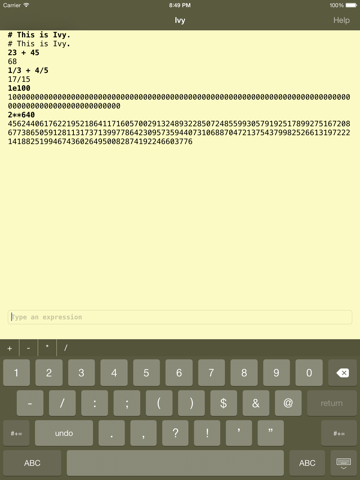Category: Utilities
Version: 1.1
Size: 4.5 MB
Free

Description

```Ivy is an experimental big number calculator.

It uses exact rational arithmetic so it can handle arbitrary precision. It also supports high precision irrational values that result from operations such as square root. Values may be input as integers, rationals, or floating point values.

FEATURES:
• Arbitrary floating point formatting
• Exact rational arithmetic
• High precision irrational arithmetic
• APL-like vector and matrix operations.
• Trigonometric, logarithmic, and exponential functions

A more complete description of the underlying engine is available at http://godoc.org/robpike.io/ivy.```

What's New in Version 1.1

`Interpreter improvements and iOS 10 support.`

Screenshots for для Айфона, Айпада